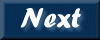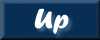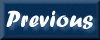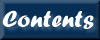Next: 8. QDP/PLT Up: XRONOS User's Guide Previous: 6. Windows   Contents

Subsections

# 7. Output File Format

The analysis results are output in a FITS file. Two different FITS layouts are available (selected with parameter outfiletype). For outfiletype=1, the file stores one interval (or frame) of interval results per FITS table row, and the output file will have a single extension. For outfiletype=2 (the default), the file stores one interval (or frame) per FITS extension, and the output file will have as many extensions as the number of intervals (or frames).

# 7.1 Statistical Keywords

A number of statistical variables (and errors if appropriate) associated with each interval (or frame) for each time series are calculated and stored as FITS keywords in the output file:

```     * `AVRGE_n  ` - Average count/s in frame (in a frame this is the average
of the averages in intervals)
* `AVRGE_En ` - Error in average
* `FREXP_n  ` - Fractional exposure in frame (from average of fractional
exposures in intervals, the latter is the ratio of good
newbins to the total number of expected newbins/interval)
* `FREXP_En ` - Error in fractional exposure
* `VAROB_n  ` - Variance in frame (average of variances in intervals)
* `VAROB_En ` - Error in variance
* `VAREX_n  ` - Expected variance in frame (average of the expected
variances in intervals, the latter is calculated from the
error bars of the newbins
* `VAREX_En ` - Error in expected variance
* `THRDM_n  ` - Third  moment in frame (average of 3rd moments in intervals)
* `MININ_n  ` - Minimum count/s in frame
* `MAXIN_n  ` - Maximum count/s in frame
* `EXVAR_n  ` - Excess variance in frame (average of excess variances in
intervals, the latter is calculated as variance-expected
variance)
* `CHI2_n   ` - Chi-square in frame (average of chi-squares in intervals)
* `CHI2_En  ` - Error in chi-square
* `RMS_n    ` - RMS fractional variation in frame (average of RMS fractional
variation in intervals, the latter is calculated as the
square-root of the excess variance divided by the average.
* `RMS_En   ` - Error in RMS
```

# 7.2 Columns

The standard output FITS files for XRONOS tasks (outfiletype=2) contain the following columns of data, documented using the form:

taskname (file extension)

```     * `COLUMN    ` - column description
```

autocor (.fac)

```     * `DELAY     ` - Time delay
* `XAX_E     ` - Half-width of time delay bin
* `AUTO_CORR ` - Auto correlation
* `ERROR     ` - Error in auto correlation
```

crosscor (.fcc)

```     * `DELAY     ` - Time delay
* `XAX_E     ` - Half-width of time delay bin
* `CROSS_CORR` - Cross correlation
* `ERROR     ` - Error in cross correlation
```

efold (.fef)

```     * `PHASE     ` - Phase
* `XAX_E     ` - Half-width of phase bin
* `RATEn     ` - Cnts/sec for series n
* `ERRORn    ` - Error in rate of series n
```
If efold is given 2 series, additional columns are written:

```     * `RATIO_12  ` - Ratio of ser2/ser1
* `RAT_E12   ` - Error in ratio of ser2/ser1
* `SUM12     ` - Sum of all (ser1+ser2)
* `SUM_E     ` - Error in sum
```
If efold is given 3 series, additional columns are written:

```     * `RATIO_12  ` - Ratio of ser2/ser1
* `RAT_E12   ` - Error in ratio of ser2/ser1
* `RATIO_23  ` - Ratio of ser3/ser2
* `RAT_E23   ` - Error in ratio of ser3/ser2
* `SUM123    ` - Sum of all (ser1+ser2+ser3)
* `SUM_E     ` - Error in sum
```
If efold is given 4 series, additional columns are written:

```     * `RATIO_12  ` - Ratio of ser2/ser1
* `RAT_E12   ` - Error in ratio of ser2/ser1
* `RATIO_34  ` - Ratio of ser4/ser3
* `RAT_E34   ` - Error in ratio of ser4/ser3
* `SUM1234   ` - Sum of all (ser1+ser2+ser3+ser4)
* `SUM_E     ` - Error in sum
```

efsearch (.fes)

```     * `PERIOD    ` - Period
* `CHISQRD1  ` - Chi-square
* `ERROR     ` - Error in chi-square
```

lcurve (.flc)

```     * `TIME      ` - Phase
* `XAX_E     ` - Half-width of time bin
* `RATEn     ` - Cnts/sec for series n
* `ERRORn    ` - Error in rate of series n
* `FRACEXP   ` - Fractional exposure
```
If lcurve is given 2 series, additional columns are written:

```     * `RATIO_12  ` - Ratio of ser2/ser1
* `RAT_E12   ` - Error in ratio of ser2/ser1
* `SUM12     ` - Sum of all (ser1+ser2)
* `SUM_E     ` - Error in sum
```
If lcurve is given 3 series, additional columns are written:

```     * `RATIO_12  ` - Ratio of ser2/ser1
* `RAT_E12   ` - Error in ratio of ser2/ser1
* `RATIO_23  ` - Ratio of ser3/ser2
* `RAT_E23   ` - Error in ratio of ser3/ser2
* `SUM123    ` - Sum of all (ser1+ser2+ser3)
* `SUM_E     ` - Error in sum
```
If lcurve is given 4 series, additional columns are written:

```     * `RATIO_12  ` - Ratio of ser2/ser1
* `RAT_E12   ` - Error in ratio of ser2/ser1
* `RATIO_34  ` - Ratio of ser4/ser3
* `RAT_E34   ` - Error in ratio of ser4/ser3
* `SUM1234   ` - Sum of all (ser1+ser2+ser3+ser4)
* `SUM_E     ` - Error in sum
```

powspec (.fps)

```     * `FREQUENCY ` - Frequency
* `XAX_E     ` - Half-width of frequency bin
* `POWER     ` - Power
* `ERROR     ` - Error in power
* `NUM_POINT ` - Number of power spectrum bins averaged in frequency
(as result of rebinning) or in time (as results of
averaging intervals in frame).
```

timeskew (.fts)

```     * `DELAY     ` - Time delay
* `XAX_E     ` - Half-width of time delay bin
* `TIME_SKEW ` - Time skewness
* `ERROR     ` - Error in time skewness
```Next: 8. QDP/PLT Up: XRONOS User's Guide Previous: 6. Windows   Contents
Alex Padgett 2009-08-21